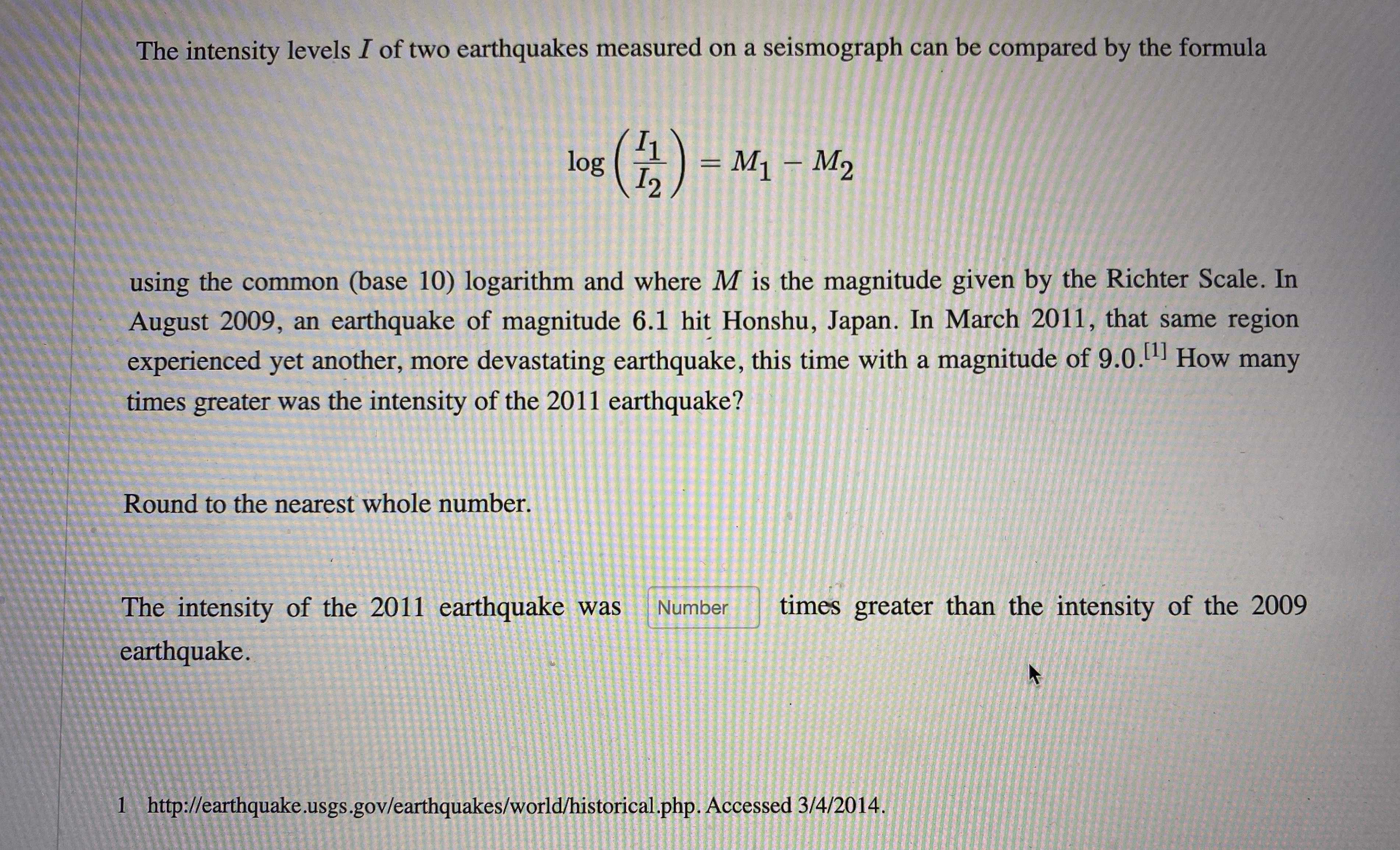### Still have math questions?

Algebra
QuestionThe intensity levels $$I$$ of two earthquakes measured on a seismograph can be compared by the formula

$$\log ( \frac { I _ { 1 } } { I _ { 2 } } ) = M _ { 1 } - M _ { 2 }$$

using the common (base 10) logarithm and where $$M$$ is the magnitude given by the Richter Scale. In August $$2009$$ , an earthquake of magnitude $$6.1$$ hit Honshu, Japan. In March $$2011$$ , that same region experienced yet another, more devastating earthquake, this time with a magnitude of $$9.0$$ - $$^ { [ 1 ] }$$ How many times greater was the intensity of the $$2011$$ earthquake? Round to the nearest whole number.